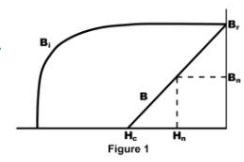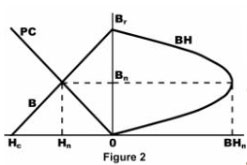• Font Size:
• A
• A
• A

## Motion Control Resources

Tech Papers

• This tech paper is filed under:
• Industry# CPUs, Sports Cars and Magnets

by Richard Stelter and Gareth Hatch, Directors of Technical Development and Technology
Dexter Magnetic Technologies, Inc.

What do users of these things have in common? An insatiable demand for maximum performance!

Computer chip manufacturers and auto manufacturers delight in the feeding frenzy that follows the announcement of a new, faster model. This “speed greed” makes premiuproducts the subject of feature review articles in industry specific media. A drop in the cost of magnet material, on a cost per unit of energy basis, has caused magnet users to join this melee, searching for the highest energy product available. More and more honest authors present the cost / performance equation in meaningful terms, suggesting that users examine their intended use before buying marginal increases in speed they cannot use. This applies to magnet-based systems also, where one must be aware of the parameters important to the function of the system.

To judge magnet parameter needs properly, one must prioritize system requirements. The key parameters include gap flux density and field uniformity under operating conditions, demagnetizing influences, and environmental factors. Generally, flux density, Bg, in the working gap of a device is of paramount importance and it can be shown by current sheet analysis that, for a given magnetic circuit, Bg is directly related to the residual flux density Br of the magnet material. Magnetic circuit geometry also determines susceptibility to operational demagnetizing factors, and the demagnetizing forces are proportional to the work extracted from the system. Environmental factors influence the total flux available and the effects of demagnetizing factors. Energy product is a design factor only if several materials are found to meet all other design requirements. To understand this, let us review how energy product is calculated.Energy product is a contraction of the term “maximum energy product”, in mega gauss-oersteds (MGOe), and the term is very descriptive of the calculation. The calculation is based on the Bn and Hn coordinates of points on the magnet’s normal operating curve, as shown in figure 1. The normal curve represents the external unit energy available from a magnet material, the energy available to the device the magnet is used in. The normal curve is directly related to the intrinsic (internal) energy curve by the formula: Bi = Bn + Hn, and extends from a point on the vertical axis that represents the residual flux density Br, where H = 0, to a point known as the coercive force Hc on the negative  horizontal axis, where B = 0. The coercive force is related to the ability of a magnet material to resist demagnetization. The value of Br is only slightly greater than Hc for most modern “straight line” high energy materials at room temperature, so the ratio of B / H, known as the recoil permeability, Ur, is just slightly greater than 1. So we can set it at 1 for the purposes of examining the energy product calculation with little error.The maximum energy product for a material is where the product of Bn · Hn peaks, as illustrated in Figure 2. At either end of the normal curve one parameter is zero, so the energy product will be zero. Selecting a fictitious material with Ur = 1.0 and Br = 12,500 G, at a Bn of 10,000 G, Hn will be 2,500 Oe and the energy product is 25,000,000 GOe. We divide by 1,000,000 and insert the prefix “mega”, so we have an energy product of 25 MGOe at this point. At Bn = 8,000 G and Hn=4,500 Oe the peak is 36 MGOe, and so on. At Bn = 6,250 G and Hn =6,250 G the energy product will be found to maximize, in this case at 39 MGOe.

Extending from this calculation, it is easy to see that, if a pure iron permanent magnet could be made with a Br of 20,000 G, the energy product would be 100 MGOe. For now, Bn seems to be asymptotic at 16,000 G because the ferrous element must be diluted by other elements to improve coercivity, so beware of promises of energy products in excess of 60 MGOe.

The maximum energy product for all high energy materials will be found to peak where the value of Bn is just slightly greater than that for Hn, and this defines another important operating characteristic, the optimum load line slope, or permeance coefficient (PC) – again, see Figure 2.

The operational PC is defined by the geometry of the magnetic circuit, and the magnet PC is defined by the geometry of the magnet prior to placing it into the circuit. The optimum use of a volume of magnet material would result if the geometry of the magnet volume resulted in the PC required to produce the material’s maximum energy product, and when inserted in the circuit produced the flux density and uniformity, and/or total flux required by the system. As a rule of thumb, the PC of the magnetic circuit will typically be close to the ratio of magnet length to working gap length. The PC for maximum energy product using modern magnet materials is close to 1.0, where Bn = 6,250 G for our fictitious material; this results from a magnet geometry with a length to (effective) diameter ratio of around 0.5. Adding to the magnetic length dimension of the magnet will improve resistance to demagnetization, so let us increase the length of the magnet by 30% so the l / d ratio is 0.65 and the PC = 1.3, where Bn = 7,065 G. If flux density in the working gap of the system is 1,000 G, then the new gap flux density would be 1,000 / 6,250 · 7,065 or 1,130 G. Note that the higher gap flux density could be achieved by increasing the area of the magnet, but this would reduce the system’s ability to resist demagnetizing forces.

For the same set of assumptions (Ur = 1.0), the Br of a 50 MGOe magnet would be 14,142 G. If one chose to replace the original 39 MGOe magnet with a 50 MGOe magnet the gap flux density would rise to 14,142 / 12,500 · 1,000 = 1,131 G, or we could reduce the diameter by 10 % to achieve a Bg of 1,000 G with the new 50 MGOe magnet.

In summary, for the example given, a 20% increase in energy product resulted in a 13% increase in gap flux density without improving the system’s ability to resist demagnetizing forces. The same increase was achieved by increasing the original magnet length by 30%, and in so doing the system’s resistance to demagnetization was improved. There are many design factors that influence the choice of a magnet material, but energy product should not be at the top of the list.